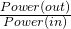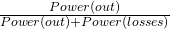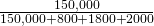Main Body

# Transformer Efficiency

While we say that transformers are very efficient, we know that they aren’t 100% efficient.

There are two main ways that transformers lose power: core losses and copper losses. Core losses are the eddy current losses and hysteresis losses of the core. They are measured using the open-circuit test. Copper losses are the I 2R losses of the primary and secondary windings. Copper losses may be measured using the short-circuit test.

## Open-Circuit Test for Core Losses

• Connect the transformer and the wattmeter, ammeter, and voltmeter as shown.
• With the primary at the rated primary voltage and the secondary open, the current running in the secondary is zero. The current in the primary will be at a minimum (roughly 2%-5% of the full-rated load).
• The current from the primary causes small circulating currents in the core. These are called eddy currents. The wattmeter is reading the heat loss in the core.

## The Short-Circuit Test for Copper Losses

• Copper losses consist of the I 2R losses in the high-voltage winding and the I 2R losses in the low-voltage winding.
• For the short-circuit test, connect the transformer as shown.
• Slowly increase the voltage until the rated current flows in the primary. Once the rated current is flowing in the primary, the rated current will flow in the secondary. The current flowing will cause heat in the windings. The wattmeter will read the winding heat losses.
• The voltmeter shows what is called the short-circuit voltage. This is the voltage that allows the rated current to flow in a short-circuit calculation. This number is used later to help determine the percent impedance of the transformer.

## Transformer Efficiency

Efficiency is always equal to the power out (Pout ) divided by the power in (Pin). Percentage efficiency (h) is:

η =x100

The power in is always equal to the power out plus the power losses (Plosses).

Power-in = Power(out)+Power(losses)

η =## Example #1

A 150 kVA transformer is tested and found to have core losses of 800 W. It also has copper losses of 1800 W in the primary coil and 2000 W in the secondary coil.

η =η =  97%